• https://pypi.org/project/xlrd/ xlrd是1.2的版本 https://pypi.org/project/xlwt/ 分别下载tar.gz 文件 解压后的文件夹，cmd python setup.py installexcel列去重
• 讲解怎么使用VBA处理 数据，主要是Excel中。包括讲解和源代码，有兴趣的可以看看--------后面描述纯属凑字数，看看即可code
• Set w = Worksheets("测试数据") Dim i As Integer, j As Integer, num As Integer, col As Integer Dim r As Range, rr As Range ' rr是需要复制的单元格 固定 For i = 4 To 10 Step 1 ' 多少行 num = ...

VBA程序如下:

Sub test1()
Dim w As Worksheet
Set w = Worksheets("测试数据")

Dim i As Integer, j As Integer, num As Integer, col As Integer
Dim r As Range, rr As Range

' rr是需要复制的单元格 固定
For i = 4 To 10 Step 1 ' 多少行
num = Range("C" & i) - 1 '复制次数
Debug.Print "复制次数" & num

col = w.Range("E" & i).End(xlToRight).Column

If col = 256 Then
Set rr = w.Range("E" & i)
Else
Set rr = w.Range(Cells(i, 5), Cells(i, col)) '确定要复制的单元格
End If

'定位最右边的第一个单元格
For j = 1 To num Step 1
Set r = w.Range("A" & i).End(xlToRight).Offset(0, 1)
rr.Copy r '复制
Next j

Next i
End Sub

得到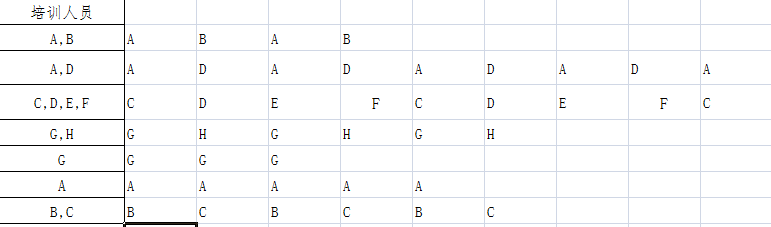然后把这些数据放在一起, 去重,  统计每个人的出现次数即可.

一列数据的去重很简单, 只需要选中这一列, 删除重复项即可. 如何做一个区域的去重?

实例(2): 区域的数据去重

原始情况如下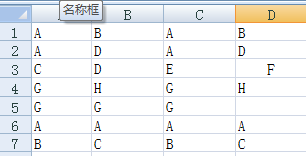VBA代码如下

Sub test2()  ' 区域去重
Dim Rng As Range, Arr, i As Long, j As Long, T As Boolean
j = 1
ReDim Arr(1 To 1)  ' arr 用来存储非重复项
T = True
For Each Rng In Selection
If Rng.Value <> "" Then
For i = 1 To j  ' j 是arr的长度, 遍历arr每一项
If Arr(i) = Rng.Value Then ' 出现重复了
Rng.Value = "" ' 删除重复的单元格内容
T = False
Exit For
End If
Next
If T Then  ' 不是重复值
j = j + 1 ' 增加数组长度
ReDim Preserve Arr(1 To j)
Arr(j) = Rng.Value ' 存储该单元格到数组中
End If
T = True
End If
Next
Range("E1:E" & j) = Application.WorksheetFunction.Transpose(Arr) ' 得到不重复项
End Sub
展开全文vba
• 筛重简单的说就是将表格中的很重复的数据进行归纳，特别是我们在选型的时候会经常用到。 在我们在设计的时候，会先把按钮啊、开关之类的先一个个的写出来，然后对应每个按钮选定一个按钮的型号，对应每个电机选定...

简要说明

之前做过用用VBA来筛选数据，去重的相关工作，但是后面给忘记了，最近需要用到，又重新写了一个。鉴于此，还是做一个归纳，以后再需要的时候也方便一些。
VBA主要用在Excel中，别的就不说了，只说Excel中的情况
一个是筛重。筛重简单的说就是将表格中的很多重复的数据进行归纳，特别是我们在选型的时候会经常用到。
在我们在设计的时候，会先把按钮啊、开关之类的先一个个的写出来，然后对应每个按钮选定一个按钮的型号，对应每个电机选定电机的型号，这样的话，在修改和查看起来就非常简单。但是在最后下采购单的时候就需要把型号一样的进行汇总，对同一个型号的列一个项目，后面做一个数量上的累加就行了。比如说，启动按钮用的是A型号，停止按钮用的也是A型号，下采购单的时候，就需要在前面列一个（名称：按钮，型号：A，数量：２）就行了，不用单独拆出来。
简要说下具体使用：

找出数据

第一步，先把需要统计的数据找出来。比如，下面一个例子：

Private Sub CommandButton2_Click()
Dim i As Integer
For i = 5 To 500
If Sheets("采购清单_test").Cells(i, "I") <> "" Then
If Sheets("采购清单_test").Cells(i, "k") = "乙供" Then
Cells(i, "D") = Sheets("采购清单_test").Cells(i, "I")
Cells(i, "C") = Sheets("采购清单_test").Cells(i, "G")
End If
End If
Next
End Sub

在上面的例子中，就是把《采购清单_test》这个表格中K里面写的是“乙供”的找出来。当然，我这个表格中的K列写的是这个零件是甲方供货还是乙方供货。在这个项目里面我们是乙方，我们就需要下标注为乙方的采购单。在新的表格里面，D列是将旧表格的第I列的数据复制过来，C列是把旧表格的G列的数据复制过来。在我的旧表格里面I列是型号，G列是数量。
经过这个程序，我们就把需要采购的东西都筛选到我的新表格中了，当然我这个是从第五行开始筛选，一直筛到第五百行，而实际上我的数据不到三百行。
因为从旧表格复制过来的数据没有进行任何处理。所以，在旧表格中是属于甲方供货的那些行就是空白行，这样就需要把空白行给剔除掉，让新的表格中的数据从上到下排列。具体的方法如下。

让数据之间没有空行

第二步，将复制过来的数据，从上往下一个挨着一个排列在一起。

Private Sub CommandButton3_Click()
Dim i, j, k, l, m, n As Integer
k = 5
n = 5
For i = 5 To 500
If Cells(i, "D") <> "" Then
Cells(k, "F") = Cells(i, "D")
Cells(k, "E") = Cells(i, "C")
k = k + 1
End If
Next
End Sub

经过这个过程，每两行的数据之间就没有空行了。

数据整合，主要目的是去重

第三步，就是对已经筛选出来的数据进行整合了。同样的型号归到同一类中。看下面的例子：

Private Sub CommandButton1_Click()
Dim i, j, k, l, m, n As Integer
k = 5
n = 5
For i = 5 To 500
Cells(i, "G") = ""
Cells(i, "H") = ""
Next
For i = 5 To 500
If Cells(i, "F") <> "" Then
m = 2
For j = 5 To 500
If Cells(j, "G") = Cells(i, "F") Then
Cells(j, "H") = Cells(j, "H") + Cells(i, "E")
m = 1
End If
Next
End If
If m = 2 Then
Cells(n, "G") = Cells(i, "F")
Cells(n, "H") = Cells(i, "E")
n = n + 1
m = 1
End If
Next
End Sub

当然，这个例子里面，就是把相同型号的放到同一行，在数量上面是相同型号的数据的总和。

展开全文编程语言 vba excel
• excel对比两边数据去重

千次阅读 2020-09-29 15:52:12
首先，A列数据要分列，因为数据格式不一样，会导致后面的问题，一直下一步到完成 第二步，在B和D列加数字1，相当于索引，相同的数据长度，做匹配，下图A和C的数据取反了 第三步，数据--筛选，然后过滤掉C...

需求:筛选重复数据，A列是1000条数据，C列是100条数据，删除重复的数据，只剩900条。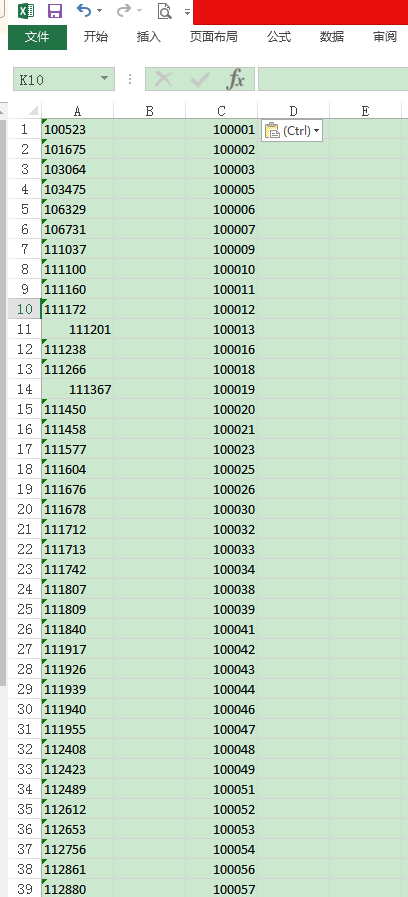首先，A列数据要分列，因为数据格式不一样，会导致后面的问题，一直下一步到完成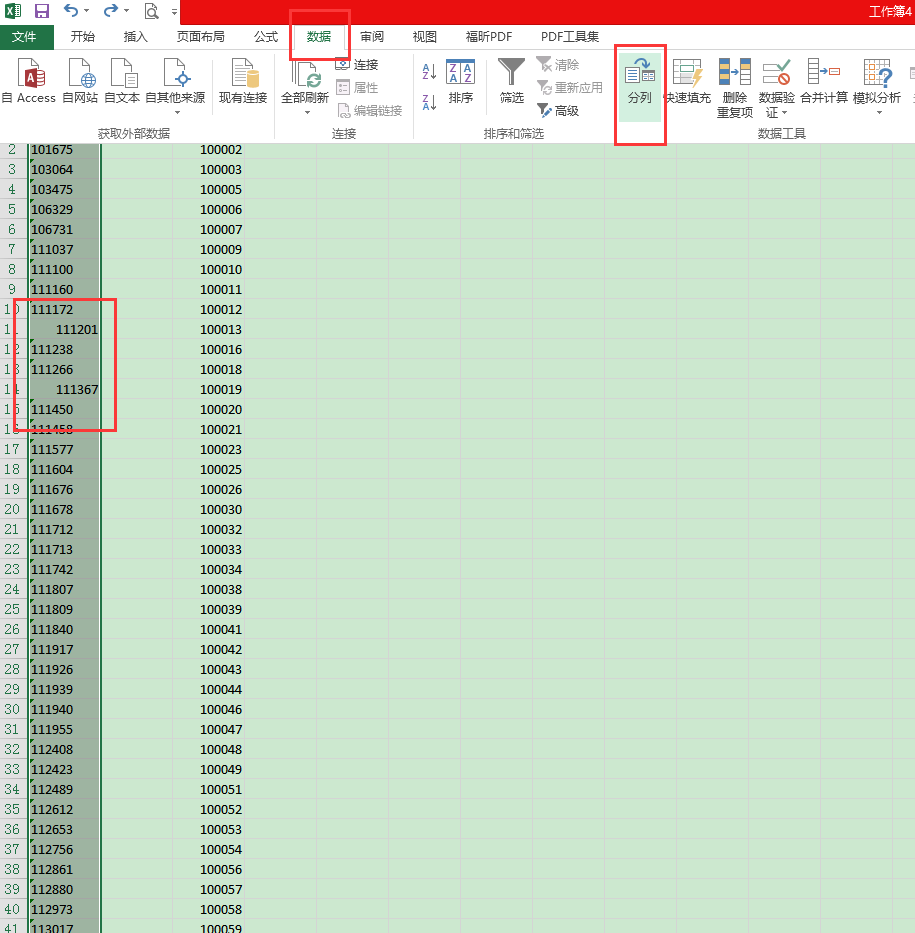第二步，在B和D列加数字1，相当于索引，相同的数据长度，做匹配，下图A和C的数据取反了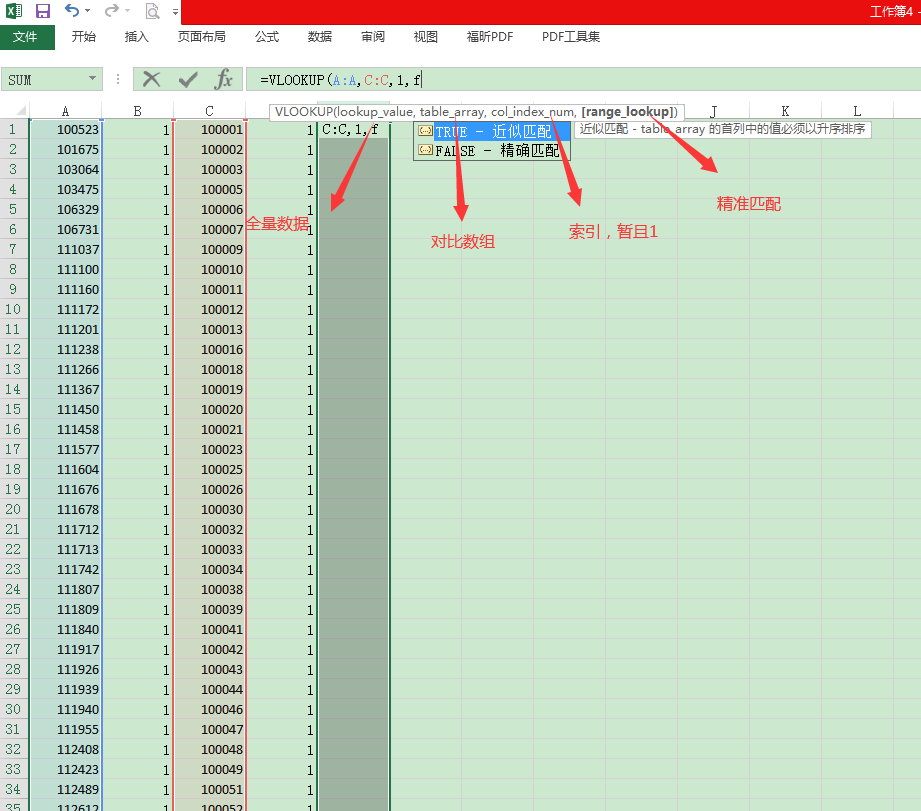第三步，数据--筛选，然后过滤掉C列数据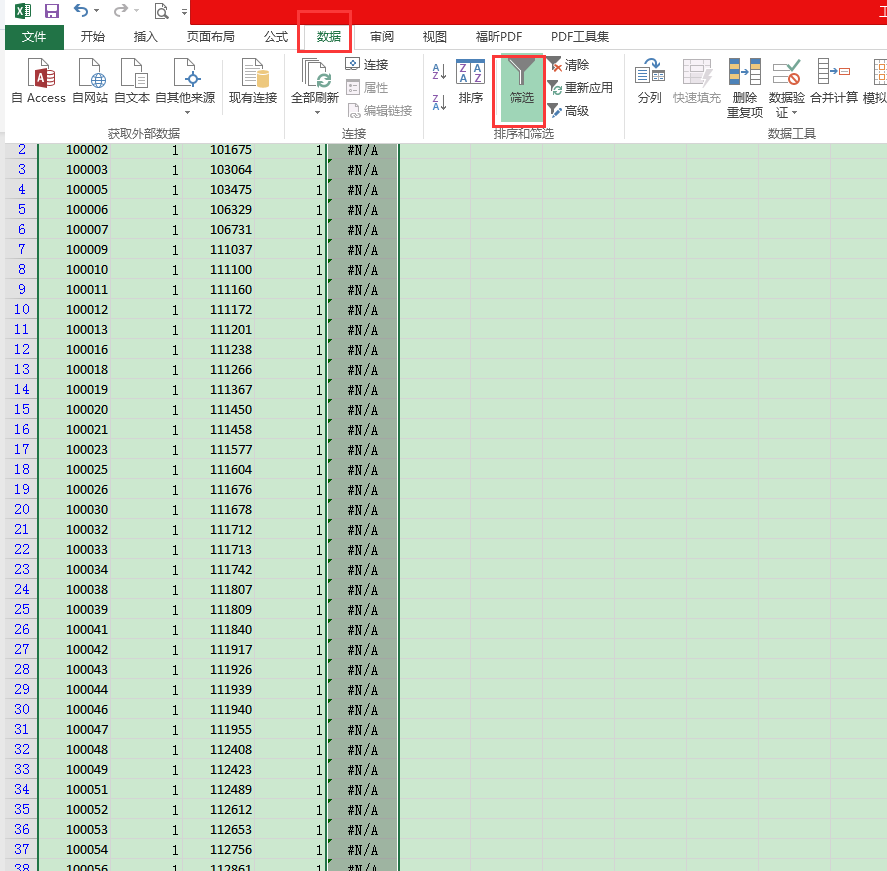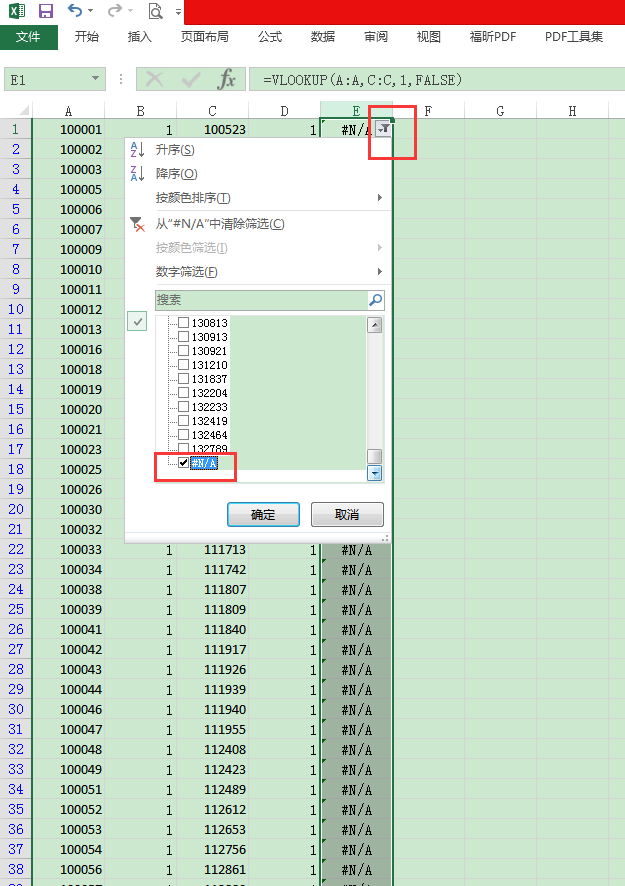最后复制A列的数据，已经处理好的数据，到其他页面，验证完成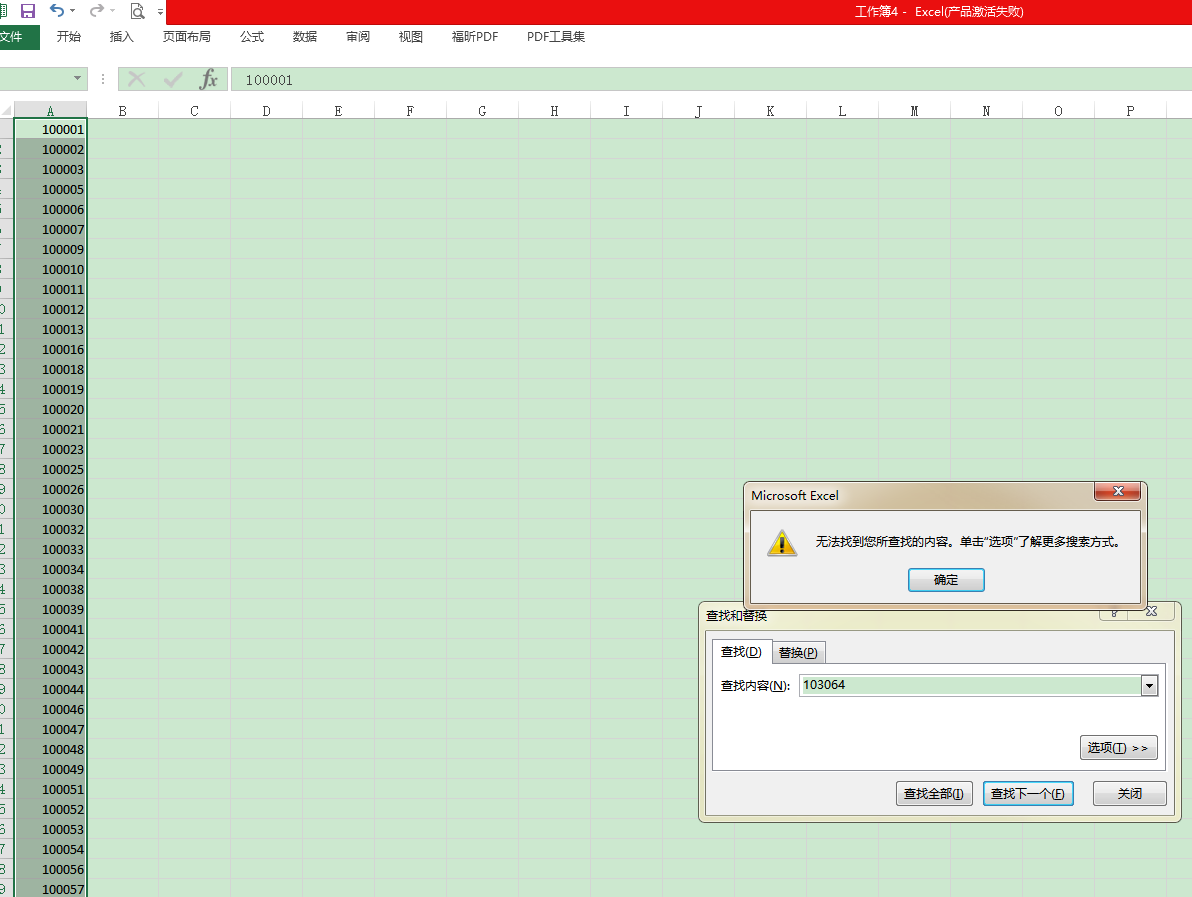展开全文• 原标题：「总结篇」Python中所有的Excel操作技巧Python对于Excel的操作是多种多样的，掌握了相关用法就可以随心所欲的操作数据了！操作xls文件xlrd（读操作）：import xlrd1、引入xlrd模块workbook=xlrd.open_...

原标题：「总结篇」Python中所有的Excel操作技巧

Python对于Excel的操作是多种多样的，掌握了相关用法就可以随心所欲的操作数据了！

操作xls文件

xlrd（读操作）：

import xlrd

1、引入xlrd模块

workbook=xlrd.open_workbook("36.xls")

2、打开[36.xls]文件，获取excel文件的workbook（工作簿）对象

names=workbook.sheet_names()

3、获取所有sheet的名字

worksheet=workbook.sheet_by_index(0)

4、通过sheet索引获得sheet对象

worksheet为excel表第一个sheet表的实例化对象

worksheet=workbook.sheet_by_name("各省市")

5、通过sheet名获得sheet对象

worksheet为excel表sheet名为【各省市】的实例化对象

nrows=worksheet.nrows

6、获取该表的总行数

ncols=worksheet.ncols

7、获取该表的总列数

row_data=worksheet.row_values(n)

8、获取该表第n行的内容

col_data=worksheet.col_values(n)

9、获取该表第n列的内容

cell_value=worksheet.cell_value(i,j)

10、获取该表第i行第j列的单元格内容

xlwt（写操作）：

import xlwt

1、引入xlwt模块

book=xlwt.Workbook(encoding="utf-8")

2、创建一个Workbook对象，相当于创建了一个Excel文件

3、创建一个sheet对象，一个sheet对象对应Excel文件中的一张表格。

sheet.write(i, j, '各省市')

4、向sheet表的第i行第j列，写入'各省市'

book.save('Data\36.xls')

5、保存为Data目录下【36.xls】文件

操作xlsx文件

openpyxl（读操作）：

import openpyxl

1、引入openpyxl模块

2、打开[36.xlsx]文件，获取excel文件的workbook（工作簿）对象

names=workbook.sheetnames

3、获取所有sheet的名字

worksheet=workbook.worksheets

4、通过sheet索引获得sheet对象

worksheet为excel表第一个sheet表的实例化对象

worksheet=workbook["各省市"]

5、通过sheet名获得sheet对象

worksheet为excel表sheet名为【各省市】的实例化对象

ws = workbook.active

6、获取当前活跃的worksheet,默认就是第一个worksheet

nrows=worksheet.max_row

7、获取该表的总行数

ncols=worksheet.max_column

8、获取该表的总列数

content_A1= worksheet['A1'].value

9、获取该表A1单元格的内容

content_A1=worksheet.cell(row=1,column=1).value

10、获取该表第1列第1列的内容

openpyxl（写操作）：

import openpyxl

1、引入openpyxl模块

workbook=openpyxl.Workbook()

2、创建一个Workbook对象，相当于创建了一个Excel文件

worksheet = workbook.active

3、获取当前活跃的worksheet,默认就是第一个worksheet

worksheet.title="test"

4、worksheet的名称设置为"test"

worksheet = workbook.create_sheet()

5、创建一个新的sheet表，默认插在工作簿末尾

worksheet.cell(i,j,'空')

6、第i行第j列的值改成'空'

worksheet["B2"]="空"

7、将B2的值改成'空'

worksheet.insert_cols(1)

8、在第一列之前插入一列

worksheet.append(["新增","台湾省"])

9、添加行

workbook.save("Data\36.xlsx")

10、保存为Data目录下【36.xlsx】文件

pandas处理excel文件

pandas操作：

import pandas as pd

1、引入pandas模块

2、读取[36.xls]或者[36.xlsx]文件

3、读取[36.csv]文件

data=data.dropna(subset=['店铺'])

4、过滤掉data店铺列有缺失的数据

data.sort_values("客户网名", inplace=True)

5、将data数据按照客户网名列进行从小到大排序

data = pd.read_csv(36.csv, skiprows = [0,1,2],sep = None, skipfooter = 4)

6、读取[36.csv]文件，前三行和后四行的数据略过

data = data.fillna('空')

7、将data中的空白处填充成'空'

data.drop_duplicates('订单','first',inplace=True)

8、data中的数据，按照【订单】列做去重处理，保留第一条数据

data=pd.DataFrame(data,columns=['订单','仓库'])

9、只保留data中【订单】【仓库】列的数据

data = data[(data[u'展现量'] > 0)]

10、只保留【展现量】列中大于0的数据

data= data[data["订单"].str.contains('000')]

11、只保留【订单】列中包含'000'的数据

data= data[data["仓库"]=='正品仓']

12、只保留【仓库】列是'正品仓'的数据

xs= data[data["店铺"]=='南极人']['销售额']

13、获取店铺是南极人的销售额数据

data['订单'] = data['订单'].str[3:7]

14、【订单】列的值只保留4-8个字节的值

data["邮资"] = np.where((data['店铺'].str.contains('T|t')) & -(data['仓库'] == '代发仓'), 8, data['邮资'])

15、满足店铺列包含 T 或 t 并且仓库不等于'代发仓'的话，将邮资的值改成8，否则值不变

data = np.array(data).tolist()

16、将data从DataFrame转换成列表

data=pd.DataFrame(data)

17、将列表转换成DataFrame格式

zhan = data[u'展现'].sum().round(2)

18、将data中所有展现列数据求和，并取两位小数

sum=data.groupby(['店铺'])['刷单'].sum()

19、将data中按照店铺对刷单进行求和

counts=data['店铺'].value_counts()

20、将data按照店铺进行计算

avg=data.groupby(['店铺'])['刷单'].mean()

21、将data按照店铺对刷单进行求平均数

count = pd.concat([counts,sum], axis=1, ignore_index=True, sort=True)

22、将counts和sum两个DataFrame进行了组合

count=count.rename(index=str, columns={0: "订单", 1: "成本"})

23、将新生成的DataFrame列名进行修改

data = pd.merge(sum, counts, how='left', left_on='店铺', right_on='店铺')

24、将两个DataFrame对象sum、counts按照店铺进行合并

data=pd.DataFrame(data)

25、将列表转换成DataFrame格式

data=pd.DataFrame(data)

26、将列表转换成DataFrame格式

data=pd.DataFrame(data)

27、将列表转换成DataFrame格式

from openpyxl import Workbook

wb=Workbook()

ws1=wb.active

data.to_excel('36.xlsx')

wb.close()

28、data完整的写入到关闭过程，执行此操作的时候【36.xlsx】不能是打开状态

excel格式操作

样式处理：

1、打开【36.xlsx】

sheet=workbook.worksheets

2、将第一个sheet对象赋值给sheet

sheet.column_dimensions['A'].width = 20.0

3、将A列的宽度设置为20

sheet.row_dismensions.height = 20.0

4、将第一行的行高设置为20

sheet.merge_cells('A1:A2')

5、将sheet表A1和A2单元格合并

sheet.unmerge_cells('A1:A2')

6、将sheet表A1和A2单元格取消合并

sheet.insert_rows(2,2)

7、将sheet表从第2行插入2行

sheet.insert_cols(3,2)

8、将sheet表从第3列插入2列

sheet.delete_rows(2)

9、删除第2行

sheet.delete_cols(3, 2)

10、将sheet表从第3列开始删除2列

from openpyxl.styles import Font, Border, PatternFill, colors, Alignment

11、分别引入字体、边框、图案填充、颜色、对齐方式

sheet.cell(i,j).font = Font(name='Times New Roman', size=14, bold=True, color=colors.WHITE)

12、设置sheet表第 i 行第 j 列的字体

sheet.cell(i,j).alignment = Alignment(horizontal='center', vertical='center')

13、设置sheet表第 i 行第 j 列的字体对齐方式

left, right, top, bottom = [Side(style='thin', color='000000')] * 4

sheet.cell(i,j).border = Border(left=left, right=right, top=top, bottom=bottom)

14、引入边框样式并调用

fill = PatternFill("solid", fgColor="1874CD")

sheet.cell(1,j).fill = fill

15、引入填充样式，并调用

结束语

总体来说用Python操作Excel的技巧大致总结了这么多，以后遇到类似的问题就可以解决了，是不是发现原来Python可以这么神奇！

———— e n d ————觉得文章不错的，欢迎点在看和转发返回搜狐，查看更多

责任编辑：

展开全文• 想把两个数据集取并集 数据——合并计算 选择好两个数据区域，确定即可。 然后就得到：excel
• EXCEL中怎么去重列重复还重复？ 追问： 整个EXCEL 回答： 按判断还是单元格判断重复？也就是说可能名字不，但是邮箱重复像这样的你算不算重复？ 追问： 按的话 只能按 按行的话 只能按行 我的意思是既在行也在...
• 怎样用python对Excel中的某些列去重？ 需求描述： 需要对表格去重，有时候是依据某一，有时候是依据某几列的组合。提供.xlsx格式，输出也要表格。 难点： 表格数据量大，几万行以上，直接用Excel常常很卡，但有时...excel python
• excel 如何将各列数据去重后合并到各列的一个单元格里如图所示，如果使excel2019或者365使用textjoin函以轻松实现=TEXTJOIN(";",,IF(MATCH(A1:A10,A1:A10,)=ROW(1:10),A1:A10,"")) 数组公式c...textjoin去重
• excel透视表计数去重 Excel数据透视表中的唯一计数 (Count Unique in Excel Pivot Table)In a previous article, Roger Govier showed us a couple of different ways to get a Unique count with a Pivot Table. ...java python 大数据 人工智能 机器学习
• 1，检查下excel表格中需要整合去重数据是否带有空格，如果带有空格必须先去除空格 首先选中需要去除空格的数据，之后单击【数据】按钮，选择【分列】，点击下一步， 点选【空格】 继续点选下一步，最后点选...excel 去重
• 20万条公司信息，每家公司对应四个行业细分字段，现需要对各个公司的四个细分行业数据进行整合，去除重复项，保留和合并不同值 ** 代码实现 ** 读写excel三方包：xlwings 注意：xlwings无法处理空值，需要预先在...set 去重
• Excel两列数据去重

万次阅读 2015-01-28 11:47:19
上次添加数据时，之前有存在相同的数据，于是要插入不存在的数据， 百度+自己研究了一番！完成，相当实用，个人感觉：原教程
• 前情提要：做图表时，希望更新数据后能自动化更新图表，需要各种公式之间相互配合。...2、构造辅助对同一用户进行排序，即统计该用户从A5开始出现的总次数(G)=COUNTIFS(A\$5:A5,A5)然后构造行号辅助，...
• 1 起因 在编写测试sql行转列的时候，需要筛选出条件数据。要对数据进行一些处理。当然可以通过使用数据库sql语句来处理，但是讲道理技不压身，因此想...现在要对prod_id这一列数据进行去重，还要在每一项前面加一...
• python中对已经生成的Series，怎样组合成DataFrame如 a = Series([1,2,3]) b = Series([2,3,4]) 怎样将a b组合成一个DataFzip函数接受任意个（包括0个和1个）序列作为参数，返回一个tuple列表。具体意思不好用文字...
• 于是去百度，但是发现好多东西百度上说的千花乱坠，但是真正可以实现的寥寥无几，于是自己又稍稍结合网上的东西以及自己的模糊记忆做了一些东西，现在将excel中的去重以及数据填充方法加以分享，愿对各位有所帮助。...office Excel Vlookup
• 数据源,如图1-1所示 ...[D:D].RemoveDuplicates Columns:=1 '对D列数据请将去重操作 End Sub 2.使用数组方式直接去重 Sub 数组去重() On Error Resume Next Dim arr1() arr = Range("A2:A21") ReDexcel vba
• Pandas 同元素多列去重的实例有一些问题可能会遇到同元素多列去重问题，下面介绍一种非常简单效率也很快的做法，用pandas来实现。首先我们看一下数据类型：G1 G2a bb ac dd ce f对这样的两列数据进行同元素去重，...
• excel vba 去重

千次阅读 2020-12-14 18:01:15
需求：把A的不重复的值放在B。 1.vba脚本： Option Explicit Sub quchong() Dim i, m As Integer i = 1 m = 1 Do While Cells(i, "a") <> Empty And Cells(i, "a") <> "" If InStr(1, ...
• excel表格把十几个小表的数据统计成一个总表的办法如下：1、打开excel表单。表单中有三个工作簿。子窗体记录汉语、数学和英语的结果。现在，这三个结果应该以一种形式总结。2、单击“摘要”工作簿选项，然后选择一个...
• 一、概述 ...个地区合并为一行，用逗号隔开 收入进行累计计算 最后将统计结果，保存到新的excel中。 二、演示 完整代码如下： # ！/usr/bin/python3 # -*- coding: utf-8 -*- import pandas as p...python java 数据分析 大数据 excel
• Excel如何去重

千次阅读 2021-06-02 09:10:55
简单的表格去重方法，可以直接在Excel软件中进行，如下图：表格工具 excel去重 表格去重 excel重复项
• excel去重函数

千次阅读 2020-12-23 10:08:32
在日常工作问题中，经常会遇到一个问题，当你呼哧呼哧处理了大半天的数据后才发现，原始数据好多重复的，导致你得到的结果全部错误……顿时，想砍人的心都有了，心理阴影面积无穷大……当然，胖斯基也遇到过，不过秉...excel函数去重
• 通过自定义窗体录入信息，用Excel VBA代码实现去重验证。文末对用到的主要知识点进行了梳理。Excel VBA 去重 重复
• 废话不说，估计只有我这个菜鸟废了2个小时才搞出来，主要是我想了太方法来实现，最后都因为这因为那的原因失败了间接说明自己对可变与不可变类型的了解，还是不够透彻最后就用了个笨方法解决了！#coding:utf-8...
• Python：这事我比你熟 数据出现重复值是我们日常工作中经常会遇到的问题，例如下面这个EXCEL表格里，订单编号这一里出现了个重复值，这个时候我们就要对数据进行去重了。去重的方法有很，这里介绍两种方法：1...
• mysql 数据去重

2021-01-19 09:52:10
excel中导入了一部分数据到mysql中，有很多数据是重复的，而且没有主键，需要按照其中已经存在某一数据进行去重。添加主键由于之前的字段中没有主键，所以需要新增一个字段，并且将其作为主键。添加一个新的...
• 网上有很方法，辅助、sql、PowerPivot插件，都太麻烦了，我还是比较懒。最后发现，其实这只是一个打个勾勾的事情： 如下截图，在建立数据透视表的时候，勾选上：添加到数据模型（Add this data to the Data ......